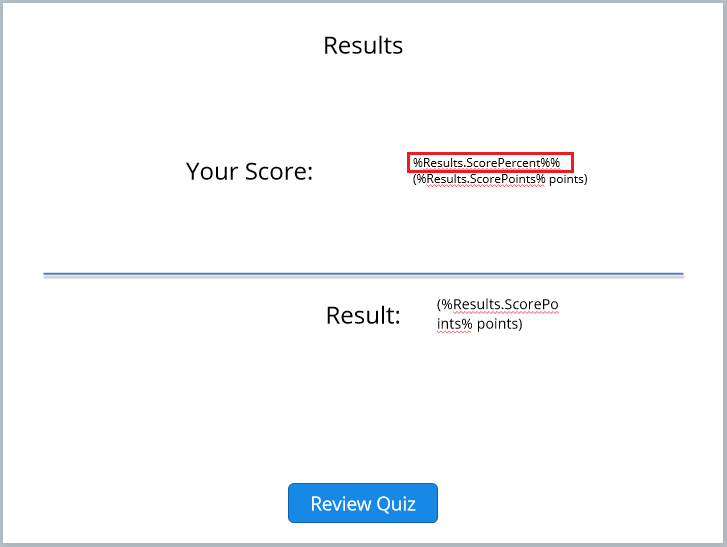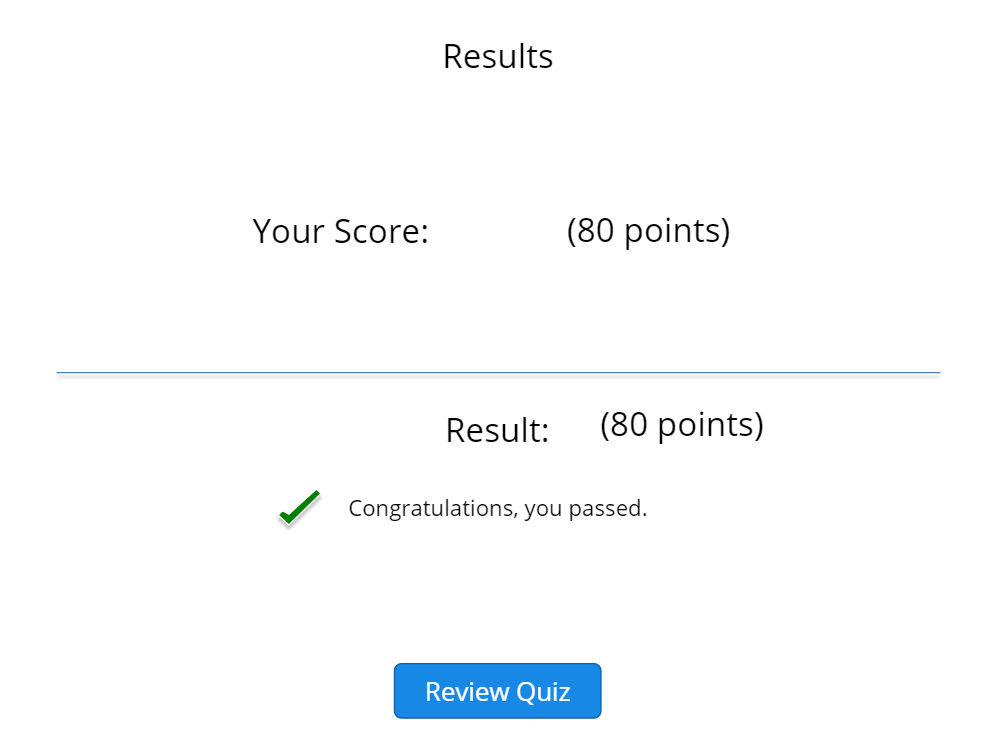How can i set score manually in graded question?

Hi,

I'd like to set score manually in graded question by choice.

For example:

I have 16 question, and each has 5 choices such as strongly disagree is 1 point, disagree is 2 point, ..., ..., strongly agree is 5 point.

If the participant choose strongly agree (5 point) in every question then user has 80 point (5 x 16 = 80), but if participant choose:
2 point for 5 question (2 x 5 = 10),
3 point for 5 question (3 x 15 = 15),
4 point for 3 question (4 x 3 = 12),
5 point for 3 question (5 x 3 = 15),
then the result is 52.

But now, in articulate, if the participant choose 5 point in each question then the return is 100%.

Could you help me how to set score 100% became 80%?

Thank you

Jufren

3 RepliesHi Jufren,

Looks like you have already assigned a corresponding score for every choice you've provided in the Graded Question you've set up.

The problem, if I understood it correctly is you want users to see the total actual score and NOT the percentage of the correct answers they earned for the quiz.

If this is the case, you need to add a result slide. Once added, you can modify which result you want the test takers to see - total points earned or percentage of the correct answers

Here's a basic example on how you can show the actual points the user earned for the quiz in the result slide. Below assumes you've already added a result slide. If you've not done it yet, feel free to visit this tutorial.

From the result slide, remove the highlighted text below.Once removed, the preview will return only the total points the test taker earned. I.E., I tried to create a basic version of the scenario you described in this post where a user earned 5 points for each question in the quiz. (5 points x 16 = 80)I hope this helps. Feel free to let me know if you're expecting something else.

Best,

Jopney